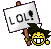# Classic Merge Sort##Classic Merge Sort

public class MergeSort{
private int[] numbers;
private int number;

public sort(int[] values){
this.numbers=values;
this.number=value.length();
mergesort(0,number-1);
}
public void mergesort(int low, int high){
if(low<high){
int mid=(low+high)/2;
mergesort(low, mid);
mergesort(mid+1,high);
merge(low,mid,high);
}
}

public void merge(int low, int mid, int high){
int[] helper=numbers;
int i=low;
int j=mid+1;
int k=low;
while(i<=mid&&j<=high){
if(helper[i]>helper[j]){
numbers[k++]=helper[j++];
else{
numbers[k++]=helper[i++];
}
}
if(i!=mid){
while(i<=mid){
numbers[k++]=helper[i++];
}
}
if(j!=mid){
while(j<=high){
numbers[k++]=helper[j++];
}
}
}
}
}

##This is a test!

This is a test#include <iostream>

using namespace std;

int main() {
cout << "Hello, world!" << endl;
}

Last edited by viterbi on Sat Sep 03, 2011 1:57 pm; edited 1 time in total

##Re: Classic Merge Sort

viterbi wrote:This is a testFirst time someone write message here!

##Re: Classic Merge Sort

Are you sure this is correct? In the last lines, it should be j!=high, right?

Also, I doubt that if (i!=mid) and if (j!=high) is needed at all. If i equals mid, one still need to append helper[mid] to numbers, right?

private int[] numbers;
private int number;

public sort(int[] values){
this.numbers=values;
this.number=value.length();
mergesort(0,number-1);
}
public void mergesort(int low, int high){
if(low<high){
int mid=(low+high)/2;
mergesort(low, mid);
mergesort(mid+1,high);
merge(low,mid,high);
}
}

public void merge(int low, int mid, int high){
int[] helper=numbers;
int i=low;
int j=mid+1;
int k=low;
while(i<=mid&&j<=high){
if(helper[i]>helper[j]){
numbers[k++]=helper[j++];
else{
numbers[k++]=helper[i++];
}
}
if(i!=mid){
while(i<=mid){
numbers[k++]=helper[i++];
}
}
if(j!=mid){
while(j<=high){
numbers[k++]=helper[j++];
}
}
}
}
}

##Re: Classic Merge Sort

I wrote a C++ program which shows that your if (i!=mid) is not needed, may even be wrong.

1____void merge_sort(int* a, int N) {
2__________if (N <= 1) return;
3__________int left = N/2;
4__________int right = N-N/2;
5__________merge_sort(a, left);
6__________merge_sort(a+left, right);
7____
8__________int *x = new int[N];
9__________for (int i=0; i<N; i++) x[i] = a[i];
10___
11_________int l = 0;
12_________int r = left;
13_________int p = 0;
14_________while (l<=left-1 && r<=N-1) {
15_______________if (x[l] < x[r]) a[p++] = x[l++];
16_______________else a[p++] = x[r++];
17_________}
18_________while (l<=left-1) a[p++] = x[l++];
19_________while (r<=N-1) a[p++] = x[r++];
20_________delete[] x;
21___}

##Re: Classic Merge Sort

Hi,

Thanks for your notice. I checked my code and found I use the shallow copy in the merge method which lead to error. This is the code I tested:

public class MergeSort{
private int[] numbers;
private int number;
private int[] copy;

public MergeSort(int a){
copy=new int[a];
}
public void sort(int[] values){
this.numbers=values;
this.number=values.length;
mergesort(0,number-1);
}

public void mergesort(int low, int high){
if(low<high){
int mid=(low+high)/2;
mergesort(low, mid);
mergesort(mid+1,high);
merge(low,mid,high);
}
}

public String toString(){
String str=new String();
str="";
for(int i=0;i<numbers.length;i++){
str+=numbers;

}

return str;
}

public void merge(int low, int mid, int high){
int[] helper=new int[number];
for(int k=0;k<=high;k++)
helper[k]=numbers[k];

int i=low;
int j=mid+1;
int k=low;
while(i<=mid&&j<=high){
if(helper[i]>helper[j]){
numbers[k++]=helper[j++];}
else{
numbers[k++]=helper[i++];
}
}

while(i<=mid){
numbers[k++]=helper[i++];
}

while(j<=high){
numbers[k++]=helper[j++];
}

helper=null;
}

public static void main(String[] args) {
int[] a={0,1,5,4,7,6,9,8};
MergeSort merge=new MergeSort(a.length);
merge.sort(a);
System.out.println(merge.toString());
}

}viterbi wrote:Are you sure this is correct? In the last lines, it should be j!=high, right?

Also, I doubt that [i]if (i!=mid)
and if (j!=high) is needed at all. If i equals mid, one still need to append helper[mid] to numbers, right?

private int[] numbers;
private int number;

public sort(int[] values){
this.numbers=values;
this.number=value.length();
mergesort(0,number-1);
}
public void mergesort(int low, int high){
if(low<high){
int mid=(low+high)/2;
mergesort(low, mid);
mergesort(mid+1,high);
merge(low,mid,high);
}
}

public void merge(int low, int mid, int high){
int[] helper=numbers;
int i=low;
int j=mid+1;
int k=low;
while(i<=mid&&j<=high){
if(helper[i]>helper[j]){
numbers[k++]=helper[j++];
else{
numbers[k++]=helper[i++];
}
}
if(i!=mid){
while(i<=mid){
numbers[k++]=helper[i++];
}
}
if(j!=mid){
while(j<=high){
numbers[k++]=helper[j++];
}
}
}
}
}

##Re: Classic Merge Sort

Hi, Just a suggestion. Your test case is too small. I used an array of 1,000,000 randomly generated numbers.

##Re: Classic Merge Sort

Thanks for your remind, I'll notice the test case later. It is really important in interview!

##Re: Classic Merge Sort